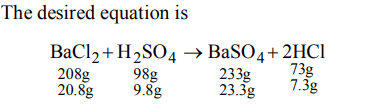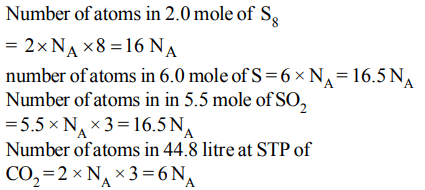## Some Basic Concepts of Chemistry Questions and Answers Part-8

1. In which of the following number all zeros are significant?
a) 0.0005
b) 0.0500
c) 50.000
d) 0.0050

Explanation: If zero is used to locate the decimal point it is considered a significant figure. In 50.000 all zero are significant

2. If law of conservation of mass was to hold true, then 20.8 gm of $BaCI_{2}$  on reaction with 9.8 gm of  $H_{2}SO_{4}$  will produce 7.3 gm of HCl and  $BaCI_{4}$  equal to :
a) 11.65 gm
b) 23.3 gm
c) 25.5 gm
d) 30.6 gm

Explanation:3. One of the following combination which illustrates the law of reciprocal proportions ?
a) $N_{2}O_{3},N_{2}O_{4},N_{2}O_{5}$
b) NaCl, NaBr, NaI
c) $CS_{2},CO_{2},SO_{2}$
d) $PH_{3},P_{2}O_{3},P_{2}O_{5}$

Explanation: In law of reciprocal proportions, the two elements combining with the third element, must combine with each other in the same ratio or multiple of that Ratio of S and O when combine with C is 2 : 1. Ratio of S and O is SO2 is 1 : 1

4. If isotopic distribution of C-12 and C-14 is 98% and 2% respectively then the no. of C-14 atoms in 12gm of carbon is
a) $1.032*10^{22}$
b) $3.0*10^{22}$
c) $5.88*10^{23}$
d) $6.02*10^{23}$

Explanation:5. Which of the following contains maximum number of atom
a) $2.0$ mole of $S_{8}$
b) 6.0 mole of S
c) $5.5$  mole of $SO_{2}$
d) $44.8$  litre of $CO_{2}$  of S.T.P.

Explanation:6. A sample of $AIF_{3}$ contains $3.0*10^{24}F^{-}$  ions. The number of formula unit of this sample are
a) $9*10^{24}$
b) $3*10^{24}$
c) $0.75*10^{24}$
d) $1.0*10^{24}$

Explanation:7. What mass of calcium chloride in grams would be enough to produce 14.35 gm of AgCl ?
a) 5.55 gm
b) 8.295 gm
c) 16.5 gm
d) 11.19 gm

Explanation:8. If potassium chlorate is 80% pure, then 48 gm of oxygen would be produced from (atomic mass of K =39)
a) $153.12$  gm of $KCIO_{3}$
b) $122.5$  gm of $KCIO_{3}$
c) $245$  gm of $KCIO_{3}$
d) $98$  gm of $KCIO_{3}$

Explanation:9. If 224 ml of a triatomic gas has a mass of 1 gm at 273K and 1 atmospheric pressure then the mass of one atom is
a) $8.30 ×10^{-23}$
b) $2.08 ×10^{-23}$
c) $5.53 ×10^{-23}$
d) $6.24 ×10^{-23}$b) $AB_{2}$
c) $AB_{3}$
d) $A_{3}B$